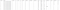# Python for Data Science — Part 1

Ordinary Least Squares vs Ridge & Lasso

## General Linear Regression

The aim here is to document machine learning fundamentals for newbies starting with supervised learning. This article focuses on general linear regression and helps overcome the problem of under/over fitting data.

This problem is then countered using ridge and lasso regularization which will be covered in detail using python programming.

Note: The ‘python for data science’ series assumes you have the basic python knowledge and know what each algorithm stands for.

First, Let’s get the required libraries in.

The dataset used is scikitlearn’s wave dataset. As mentioned earlier, the aim is to…

# Understanding MLOps — Initiating the uninitiated

## Create reproducible ML pipelines & automate the ML lifecycle

There is rarely “one pipeline” to manage an E2E process. And there is rarely “one article” to understand a new concept.

# High Level Overview

Understanding MLOps & deciphering jargon such as CI/CD/CT, automation, & deployment rely heavily on the context of our workflow architecture. Deploying a machine learning model into production can involve multiple pipelines that contribute to one large data science workflow. For instance, a Data Engineer prepares the data by sourcing it from a data lake and this is absorbed into the data catalog. This is then fed to the Data Scientist who trains the model, evaluates & tunes it before…

# Gradient Boosted Tree Regression — Spark & Python

## An overview of GBTR using pyspark & databricks — Machine Learning

This story demonstrates the implementation of a “gradient boosted tree regression” model using python & spark machine learning. The dataset used is “bike rental info” from 2011–2012 in the capital bike share system. Our goal is to predict the count of bike rentals.

# 1. Load the data

The data in store is a CSV file. We are to create a spark data frame containing the bike data set. We cache this data so that we read it only once from the disk.

`#load the dataset & cachedf = spark.read.csv("/databricks-datasets/bikeSharing/data-001/hour.csv", header="true", inferSchema="true")df.cache()df.cache()#view the imported datasetdisplay(df)`

## Output:Image by Author

# 2. Pre-Process the data

Fields such as “weekday” are indexed…

# A brief

The transition from traditional ML to spark ML involves a learning curve, but it is intriguingly adaptive. The world of pySpark has evolved that way, to say the least.

PS: The code snippets below will not include result sets, but you should be able to manipulate it with your data. Let’s jump right in:

## Why clustering?

So we can get meaningful groups from our data (unlabeled). Hence the term “unsupervised”. In this case, I’ve used the silhouette with squared euclidean distance to score the clusters.

`from pyspark.ml.clustering import KMeansfrom pyspark.ml.evaluation import ClusteringEvaluatorcol1_col2 = df.groupBy("col1", "col2").count()display(col1_col2).head()`

Assuming col1 & col2…

# Linear Regression for Data Science

## Intro to linear regression & the math behind it

Regression is the study of dependence — A Predictive modelling technique

• It attempts to find the relationship between a DEPENDENT variable “Y” and an INDEPENDENT variable “X”.
• (Note: Y should be a continuous variable while X can be categorical or continuous)
• There are two types of regression — Simple Linear Regression and Multiple Linear Regression.
• Simple linear regression will have one independent variable (predictor).
• Multiple linear regression will have more than one independent variable (predictors).
• In a nutshell — Linear Regression maps a continuous X to a continuous Y.

## 1. APPLICATIONS OF REGRESSION ANALYSIS:

1. To determine strength of independent variables (predictors)

Example: Relationship between…## Ranjith Menon

Data Scientist @ Mindcurv | Machine Learning Graduate | Masters in Information Systems @ Monash University | https://github.com/ranjithhmenon